This is “Alternative Depreciation Patterns and the Recording of a Wasting Asset”, section 10.4 from the book Business Accounting (v. 2.0). For details on it (including licensing), click here.

For more information on the source of this book, or why it is available for free, please see the project's home page. You can browse or download additional books there. To download a .zip file containing this book to use offline, simply click here.

Has this book helped you? Consider passing it on:
Creative Commons supports free culture from music to education. Their licenses helped make this book available to you.
DonorsChoose.org helps people like you help teachers fund their classroom projects, from art supplies to books to calculators.

## 10.4 Alternative Depreciation Patterns and the Recording of a Wasting Asset

### Learning Objectives

At the end of this section, students should be able to meet the following objectives:

1. Explain the justification for accelerated methods of depreciation.
2. Compute depreciation expense using the double-declining balance method.
3. Realize that the overall impact on net income is not affected by a particular cost allocation pattern.
4. Describe the units-of-production method, including its advantages and disadvantages.
5. Explain the purpose and characteristics of MACRS.
6. Compute depletion expense for a wasting asset such as an oil well or a forest of trees.
7. Explain the reason that depletion amounts are not directly recorded as an expense.

## Accelerated Depreciation

Question: Straight-line depreciation certainly qualifies as systematic and rational. The same amount of cost is assigned to expense during each period of an asset’s use. Because no specific method is required by U.S. GAAP, do companies ever use other approaches to create different allocation patterns for depreciation? If so, how are these methods justified?

Answer: The most common alternative to the straight-line method is accelerated depreciationAny method of determining depreciation that assigns larger expenses to the initial years of an asset’s service and smaller expenses to the later years; it is often justified by the assumption that newer assets generate more revenues than older assets do., which records a larger expense in the initial years of an asset’s service. The primary rationale for this pattern is that property and equipment frequently produce higher amounts of revenue earlier in their lives because they are newer. The matching principle would suggest that recognizing more depreciation in these periods is appropriate to better align the expense with the revenues earned.

A second justification for accelerated depreciation is that some types of property and equipment lose value more quickly in their first few years than they do in later years. Automobiles and other vehicles are a typical example of this pattern. Consequently, recording a greater expense in the initial years of use is said to better reflect reality.

Over the decades, a number of formulas have been invented to mathematically create an accelerated depreciation pattern—high expense at first with subsequent cost allocations falling each year throughout the life of the property. The most common is the double-declining balance method (DDB)A common accelerated depreciation method that computes expense each year by multiplying the asset’s net book value (cost less accumulated depreciation) times two divided by the expected useful life.. When using DDB, annual depreciation is determined by multiplying the current net book value of the asset times two divided by the expected years of life. As the net book value drops, the annual expense drops. This formula has no internal logic except that it creates the desired pattern, an expense that is higher in the first years of operation and less after that. Although residual value is not utilized in this computation, the final amount of depreciation recognized must be manipulated to arrive at this ending balance.

Depreciation for the building bought above for \$600,000 with an expected five-year life and a residual value of \$30,000 is calculated as follows if DDB is applied. Note how different this cost allocation pattern is than that shown in Figure 10.2 "Building Acquisition and Straight-Line Depreciation" using the straight-line method.

(cost – accumulated depreciation) × 2/expected life = depreciation expense for period

Year One:

(\$600,000 – \$0) = \$600,000 × 2/5 = \$240,000 depreciation expense

Year Two:

(\$600,000 – \$240,000) = \$360,000 × 2/5 = \$144,000 depreciation expense

Year Three:

(\$600,000 – \$384,000) = \$216,000 × 2/5 = \$86,400 depreciation expense

Year Four:

(\$600,000 – \$470,400) = \$129,600 × 2/5 = \$51,840 depreciation expense

Year Five:

(\$600,000 – \$522,240) = \$77,760,

so depreciation for Year Five must be set at \$47,760 to reduce the \$77,760 net book value to the expected residual value of \$30,000.

Note that the desired expense pattern for accelerated depreciation has resulted. The expense starts at \$240,000 and becomes smaller in each subsequent period as can be seen in the adjusting entries prepared in Figure 10.6 "Building Acquisition and Double-Declining Balance Depreciation".

Figure 10.6 Building Acquisition and Double-Declining Balance Depreciation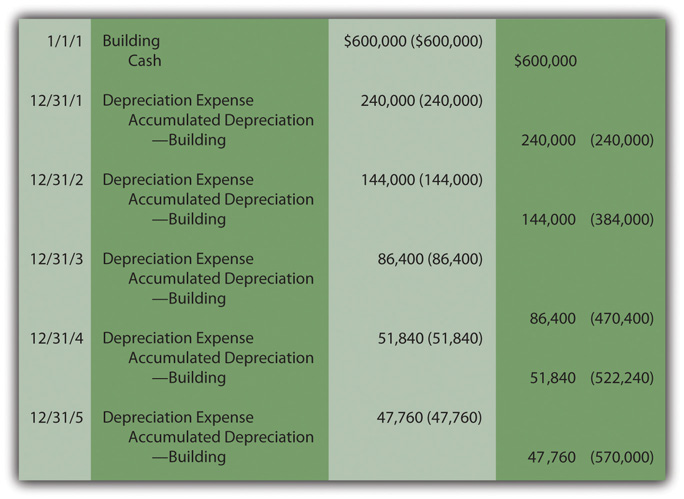When applying an accelerated depreciation method, net book value falls quickly at first because of the high initial expense levels. Thus, if an asset is sold early in its life, a reported gain is more likely (the amount received will be greater than this lower net book value). For example, in Figure 10.3 "Sale of Building at a Loss", the building was depreciated using the straight-line method and sold after two years for \$290,000, creating a reported \$82,000 loss because the net book value was \$372,000. Net book value was high in comparison to the amount received.

With DDB, the accumulated depreciation will be \$384,000 after two years as shown in Figure 10.6 "Building Acquisition and Double-Declining Balance Depreciation". If the building is then sold on December 31, Year Two for \$290,000, a \$74,000 gain is reported because net book value has dropped all the way to \$216,000 (\$600,000 cost less \$384,000 accumulated depreciation). Accelerated depreciation creates a lower net book value, especially in the early years of ownership.

Figure 10.7 Building Sold after Two Years—Double-Declining Balance Method Used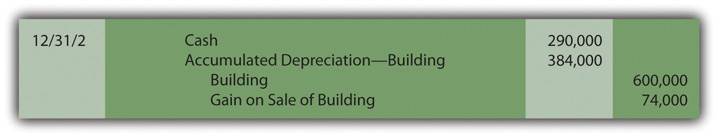Although the annual amounts are quite different, the overall net income is never affected by the allocation pattern. In this example, a building was bought for \$600,000 and later sold after two years for \$290,000. Thus, net income for the entire period of use must be reduced by the \$310,000 difference regardless of the approach applied.

Figure 10.8 Depreciation Methods—Overall Impact on Net Income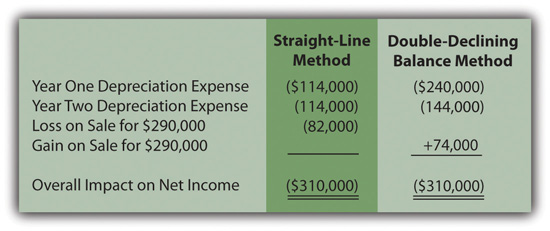### Test Yourself

Question:

On April 1, Year One, Hayden Corporation buys machinery for \$30,000 and pays an additional \$4,000 to have it delivered to its factory and then assembled. Company officials believe this machinery will last for ten years and then have a \$2,000 remaining residual value. Depreciation is to be computed by applying the double-declining balance method. The half-year convention is utilized. The value of the asset actually declines at only a rate of 10 percent per year. On the company’s balance sheet as of December 31, Year Three, what is reported as the net book value for this asset?

1. \$18,432
2. \$18,496
3. \$19,584
4. \$25,500

Answer:

The correct answer is choice c: \$19,584.

Explanation:

Cost of the asset is \$34,000, which is multiplied by 2/10 to get \$6,800. Because of the half-year convention, \$6,800 is multiplied by 1/2. Depreciation is \$3,400. For Year Two, book value is \$30,600 (\$34,000 less \$3,400). When multiplied by 2/10, depreciation is \$6,120. The contra account rises to \$9,520 and book value falls to \$24,480. When multiplied by 2/10, expense for the third year is \$4,896. Accumulated depreciation is now \$14,416 and book value is \$19,584 (\$34,000 less \$14,416).

## Units-Of-Production Method

Question: The two methods demonstrated so far for establishing a depreciation pattern are based on time, five years to be precise. In most cases, though, it is the physical use of the asset rather than the passage of time that is actually relevant to this process. Use is the action that generates revenues. How is the depreciation of a long-lived tangible asset determined if usage can be measured?

For example, assume that a limousine company buys a new vehicle for \$90,000 to serve as an addition to its fleet. Company officials expect this limousine to be driven for 300,000 miles and then have no residual value. How is depreciation expense determined each period?

Answer: Depreciation does not have to be based on time; it only has to be computed in a systematic and rational manner. Thus, the units-of-production method (UOP)A method of determining depreciation that is not based on the passage of time but rather on the level of actual usage during the period. is another alternative that is occasionally encountered. UOP is justified because the periodic expense is matched with the work actually performed. In this illustration, the limousine’s depreciation is based on the total number of miles expected. Then, the annual expense is computed using the number of miles driven in a year, an easy figure to determine.

(\$90,000 less \$0)/300,000 miles = \$0.30 per mile

Depreciation is recorded at a rate of \$0.30 per mile. The depreciable cost basis is allocated evenly over the miles that the vehicle is expected to be driven. UOP is a straight-line method but one that is based on usage (miles driven, in this example) rather than years. Because of the direct connection between the expense allocation and the work performed, UOP is a very appealing approach. It truly mirrors the matching principle. Unfortunately, measuring the physical use of most assets is rarely as easy as with a limousine.

To illustrate, assume this vehicle is driven 80,000 miles in Year One, 120,000 miles in Year Two, and 100,000 miles in Year Three, depreciation will be \$24,000, \$36,000, and \$30,000 when the \$0.30 per mile rate is applied. The annual adjusting entries are shown in Figure 10.9 "Depreciation—Units-of-Production Method".

Figure 10.9 Depreciation—Units-of-Production Method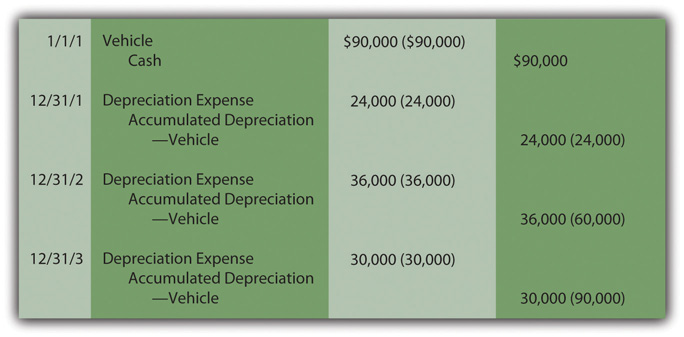Estimations rarely prove to be precise reflections of reality. This vehicle will not likely be driven exactly 300,000 miles. If used for less and then retired, both the cost and accumulated depreciation are removed. A loss is recorded equal to the remaining net book value unless some cash or other asset is received. If driven more than the anticipated number of miles, depreciation stops at 300,000 miles. At that point, the cost of the asset will have been depreciated completely.

Although alternative methods such as the double-declining balance method and the units-of-production method are interesting theoretically, they are not very widely used in practice. Probably only about 5–10 percent of companies apply a method other than straight-line depreciation. However, as indicated later, accelerated depreciation is an important method when computing a company’s taxable income.

### Test Yourself

Question:

On March 1, Year One, the Good Eating Company buys a machine to make donuts so that it can expand its menu. The donut maker cost the company \$22,000 but has an expected residual value of \$4,000. Officials believe this machine should be able to produce 100,000 dozen donuts over a ten-year period. In Year One, 7,000 dozen donuts are prepared and sold while in Year Two, another 11,000 dozen are made. However, the company is not satisfied with the profits made from selling donuts. Thus, on April 1, Year Three, after making 3,000 more dozen, the company sells the machine for \$19,000 in cash. If the units-of-production method is used to compute depreciation, what gain or loss should the company recognize on the sale of the donut maker?

1. Gain of \$780
2. Gain of \$320
3. Loss of \$320
4. Loss of \$780

Answer:

The correct answer is choice a: Gain of \$780.

Explanation:

The company expects to recognize an expense of \$18,000 (\$22,000 less \$4,000) as it makes 100,000 dozen. That is \$0.18 per dozen (\$18,000/100,000 dozen). While being used, 21,000 dozen donuts are prepared (7,000 + 11,000 + 3,000). At \$0.18 per dozen, total depreciation of \$3,780 is recognized (21,000 dozen at \$0.18 each). This expense reduces book value to \$18,220 (\$22,000 less \$3,780). If sold for \$19,000, the company reports a gain of \$780 based on the excess received (\$19,000 less \$18,220).

## MACRS

Question: Companies use straight-line depreciation, accelerated depreciation, or units-of-production depreciation for financial reporting. What method of determining depreciation is applied when a business files its federal income tax return? Can one of these methods be selected or is a specific approach required?

Answer: In most cases, the government wants businesses to buy more machinery, equipment, and the like because such purchases help stimulate the economy and create jobs. Consequently, for federal income tax purposes, companies are required to use a designed method known as Modified Accelerated Cost Recovery System (MACRS). MACRS has several built-in tax incentives inserted to encourage businesses to acquire more depreciable assets. Greater depreciation expense is allowed, especially in the earlier years of use, so that the purchase reduces tax payments.

• Each depreciable asset must be placed into one of eight classes based upon its type and life. For example, a light truck goes into one class, but a typewriter is placed in another. Every asset within a class is depreciated by the same method and over the same life.
• For most of these classes, the number of years is relatively short so that the benefit of the expense is received quickly for tax purposes.
• Residual value is ignored completely. The entire cost can be expensed.
• For six of the eight classes, accelerated depreciation is required, which again creates more expense in the initial years.

## Determining Depletion

Question: The cost of land is not depreciated because it does not have a finite life. However, land is often acquired solely for the natural resources that it might contain such as oil, timber, gold or the like. As the oil is pumped, the timber harvested or the gold extracted, a portion of the value is physically separated from the land. How is the reported cost of land affected when its natural resources are removed?

Answer: Oil, timber, gold, and the like are known as “wasting assets.” They are taken from land over time, a process referred to as depletionA method of allocating the cost of a wasting asset (such as a gold mine or an oil well) to expense over the periods during which the value is removed from the property.. Value is literally removed from the asset rather than being consumed through use as with the depreciation of property and equipment. The same mechanical calculation demonstrated above for the units-of-production (UOP) method is applied. The 2010 financial statements for Alpha Natural Resources state that “costs to obtain coal lands and leased mineral rights are capitalized and amortized to operations as depletion expense using the units-of-production method.”

Because the value is separated rather than used up, depletion initially leads to the recording of inventory (such as oil or gold, for example). An expense is recognized only at the eventual point of sale.

As with other types of property and equipment, historical cost is the sum of all normal and necessary expenditures to get the wasting asset into condition and position to generate revenues. To illustrate, assume that at the beginning of Year One, land is acquired for \$1.6 million cash while another \$400,000 is spent to construct a mining operation. Total cost is \$2 million. The land is estimated to hold ten thousand tons of ore to be mined and sold. The land will be worth an estimated amount of only \$100,000 after all ore is removed. Depletion is \$190 per ton ([\$2,000,000 cost less \$100,000 residual value]/10,000 tons). It is a straight-line approach based on tons and not years, an allocation that follows the procedures of the units-of-production method.

Assume that 3,000 tons of ore are extracted in Year One and sold in Year Two for \$1 million cash. Another 3,600 tons are removed in the second year for sale at a later time. Depletion is \$570,000 in Year One (\$190 × 3,000 tons) and \$684,000 in Year Two (\$190 × 3,600 tons). Note in Figure 10.10 "Depletion of Wasting Asset" that the ore is initially recorded as inventory and only moved to expense (cost of goods sold) when sold in the subsequent period. For depreciation, expense is recognized immediately as the asset’s utility is consumed. With depletion, no expense is recorded until the inventory is eventually sold.

Figure 10.10 Depletion of Wasting Asset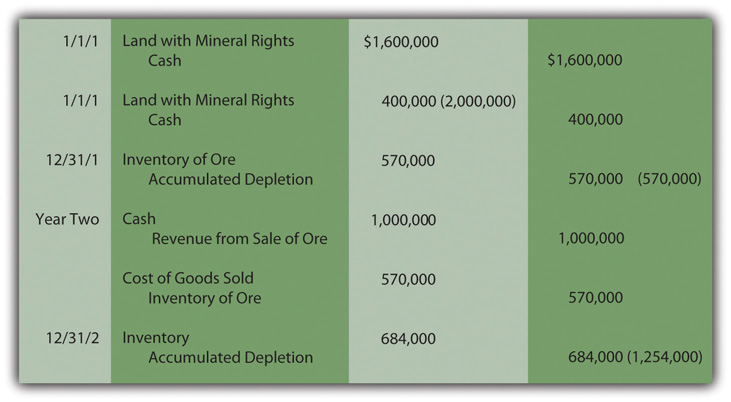After two years, this land is reported on the company’s balance sheet at a net book value of \$746,000 based on its historical cost of \$2 million and accumulated depletion to date of \$1,254,000 (\$570,000 + \$684,000). The remaining inventory of ore is reported as an asset at \$684,000 because it has not yet been sold.

Figure 10.11 Net Book Value of Land with Mineral Rights After Depletion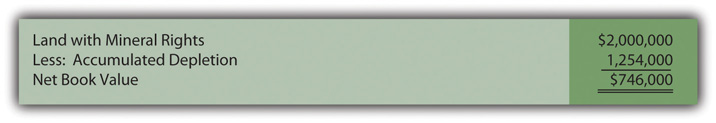### Test Yourself

Question:

When crude oil is selling for \$30 per barrel, the Ohio Oil Company buys an acre of land for \$3.2 million because it believes that a reserve of oil lies under the ground. The company spends another \$500,000 to set up the proper drilling apparatus. The company believes that 200,000 barrels of oil are in the ground at this location. Once the oil has been extracted, the land will have a resale value of only \$100,000. In both Years One and Two, 70,000 barrels are pumped out but only 50,000 are sold in Year One and another 40,000 in Year Two. The price of crude oil stays steady in Year One but jumps to \$39 per barrel during Year Two. What is the net book value of the land account at the end of Year Two?

1. \$1,080,000
2. \$1,180,000
3. \$1,740,000
4. \$2,340,000

Answer:

The correct answer is choice b: \$1,180,000.

Explanation:

The cost of this property is \$3.7 million (\$3.2 million plus \$500,000). Expected residual value is \$100,000 so \$3.6 million will be the total depletion (\$3.7 million minus \$100,000). The land holds an estimated 200,000 barrels; thus, the depletion rate is \$18 per barrel (\$3.6 million/200,000 barrels). Over the two years, 140,000 barrels are extracted so that total depletion is \$2.52 million (\$18 × 140,000). Net book value is reduced to \$1.18 million (\$3.7 million less \$2.52 million).

### Key Takeaway

Additional cost allocation patterns for determining depreciation exist beyond the straight-line method. Accelerated depreciation records more expense in the earlier years of use than in later periods. This pattern is sometimes considered a better matching of expenses with revenues and closer to actual drops in value. The double-declining balance method is the most common version of accelerated depreciation. Its formula was designed to create the appropriate allocation pattern. The units-of-production method can be used for property and equipment where the quantity of work performed is easily monitored. This approach is also used in recording the depletion of wasting assets such as oil wells and silver mines. For federal income tax purposes, the Modified Accelerated Cost Recovery System (MACRS) is required. It provides certain benefits for the acquisition of depreciable assets as a way of encouraging more purchases to help the economy grow. For example, residual values are ignored and the expense is computed for most assets using accelerated depreciation methods.